# Voltage Substractor simple circuit

• Helena Wells
Helena Wells
I have created a voltage adder using this circuit where Va and Vb are the input voltages.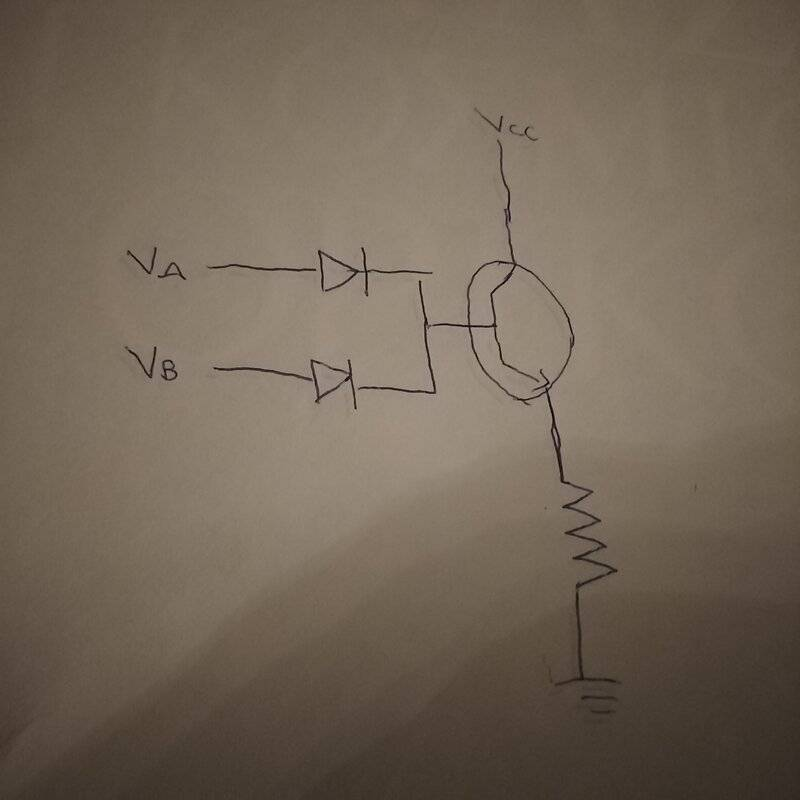And Vout is between the emitter and the resistor. How can I modify it to a voltage substractor?

Mentor
You show an Emitter Follower configuration there, and yes the voltage gain is positive (and close to 1) through it.

Have you looked the "Common Emitter" configuration with an NPN transistor yet? The gain through that circuit is negative. Have a look at CE circuits, and learn how to set the voltage gain close to negative 1.

And a step better is to start learning opamp circuits. There you can get much more accurate gain control and biasing for things like adders and subtractors.••DaveE and etotheipi
Helena Wells
You show an Emitter Follower configuration there, and yes the voltage gain is positive (and close to 1) through it.

Have you looked the "Common Emitter" configuration with an NPN transistor yet? The gain through that circuit is negative. Have a look at CE circuits, and learn how to set the voltage gain close to negative 1.

And a step better is to start learning opamp circuits. There you can get much more accurate gain control and biasing for things like adders and subtractors.This is just a common emitter amplifier with 2 inputs instead of 1.

What you have drawn is a primitive "OR" gate (if one input is "high", the output is "high". Here is a schematic of a "NOR" gate (common emitter instead of common collector).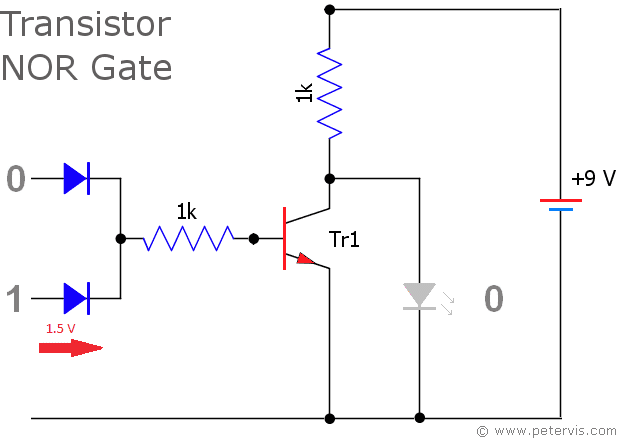Helena Wells
What you have drawn is a primitive "OR" gate (if one input is "high", the output is "high". Here is a schematic of a "NOR" gate (common emitter instead of common collector).
View attachment 267043
Actually 2 inputs with 2 voltages are supposed to increase base current even more than 1 voltage source - > more collector current and less voltage drop on the transistor so Vout will be close to Vcc.

Mentor
This is just a common emitter amplifier with 2 inputs instead of 1.
No, you've drawn a "Common Collector" or Emitter Follower configuration. The Common Emitter configuration is different: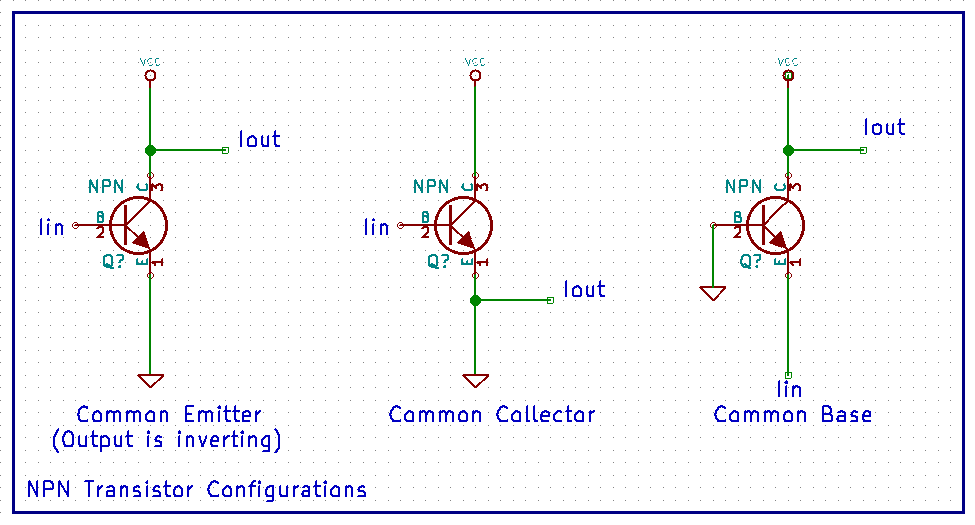•etotheipi
Mentor
Yes I know but I don't want op amps involved.Only common emitter amplifiers.
CE amps are inverting (voltage gain is negative), and Emitter Follower amps are non-inverting. If you really want to build a subtractor, you need at least one part of that circuit to be inverting, no? And the other part to be ________Mentor
I don't agree see the Common Collector amplifier has a slightly different construction.
How so?

Helena Wells
Will this work?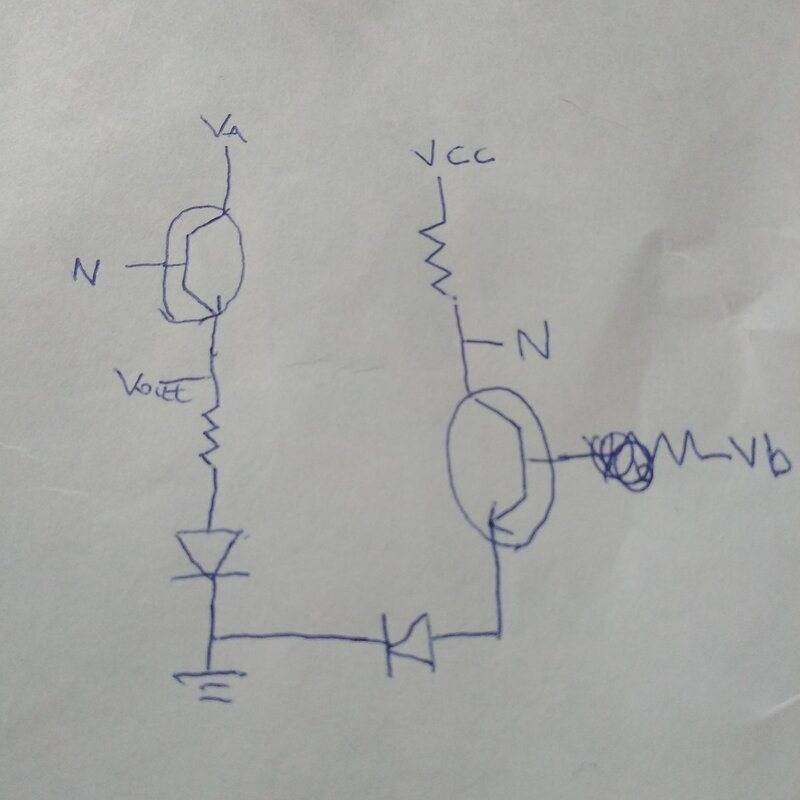Helena Wells
How so?
What you have drawn is an inverter not a common collector amplifier.

Yes I know but I don't want op amps involved.Only common emitter amplifiers.
Voltages can be converted to currents by resistors.
Current Mirrors use pairs of common emitter transistors to add and subtract currents.

Would you consider using current mirrors to make the voltage arithmetic circuit.
https://en.wikipedia.org/wiki/Current_mirror#Circuit_realizations_of_current_mirrors

If you can define the arithmetic problem, I can design a mirror.

•DaveE and hutchphd
Helena Wells
Hmm ok.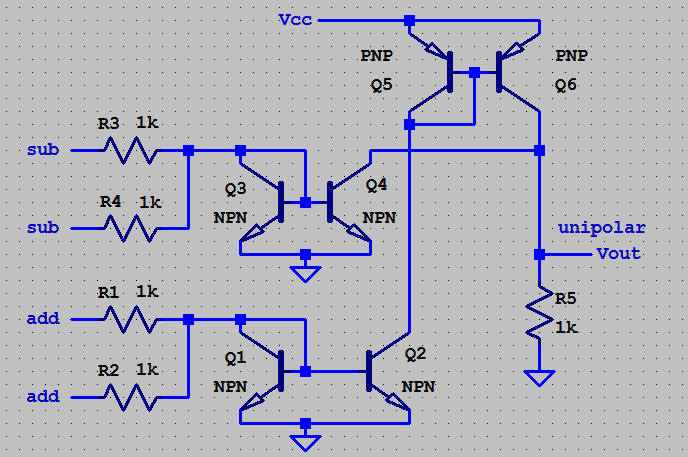•berkeman
Helena Wells
The current that flows through Q1 will mirror through Q2, because their base and emitter voltages are the same.
The current that flows through Q3 will mirror through Q4.
The current that flows through Q5 will mirror through Q6.

Q1 is the total summing currents. Q1, Q2, Q5, Q6 are all equal.
Q3 is the total subtracting currents. Q3 and Q4 are equal.

The Q4 current is removed from the collector of Q6 before the remainder flows through R5.
The output voltage is developed across R5.

Do you have a specific question?

Helena Wells
The current that flows through Q1 will mirror through Q2, because their base and emitter voltages are the same.
The current that flows through Q3 will mirror through Q4.
The current that flows through Q5 will mirror through Q6.

Q1 is the total summing currents. Q1, Q2, Q5, Q6 are all equal.
Q3 is the total subtracting currents. Q3 and Q4 are equal.

The Q4 current is removed from the collector of Q6 before the remainder flows through R5.
The output voltage is developed across R5.

Do you have a specific question?
I want to substract voltages.

If you put voltage X on a sum input and voltage Y on a sub input,
then the output voltage will be X - Y.

Actually 2 inputs with 2 voltages are supposed to increase base current even more than 1 voltage source - > more collector current and less voltage drop on the transistor so Vout will be close to Vcc.
1. If you want an addition circuit, remove the diodes (they turn the analog circuit into a digital)
2. If you want to subtract, invert the signal you want to subtract and then add (a standard common-emitter circuit will invert the signal). Figure out:
1. How to ensure that the gain of the inverter is 1.0
2. How to ensure that the DC level of the inverted signal is the same as the non-inverted.

Helena Wells
If you put voltage X on a sum input and voltage Y on a sub input,
then the output voltage will be X - Y.
OK.

Helena Wells
1. If you want an addition circuit, remove the diodes (they turn the analog circuit into a digital)
2. If you want to subtract, invert the signal you want to subtract and then add (a standard common-emitter circuit will invert the signal). Figure out:
1. How to ensure that the gain of the inverter is 1.0
2. How to ensure that the DC level of the inverted signal is the same as the non-inverted.
If I remove the diodes current may flow from 1 input to a second input and I don't want that.And I have posted another circuit which makes the subtraction.

If I remove the diodes current may flow from 1 input to a second input and I don't want that.
Then isolate the input voltages using emitter followers.

Helena Wells
Then isolate the input voltages using emitter followers.
And make it even more complex? No thank you! Simplicity is king.

Homework Helper
2022 Award
The circuit you originally proposed will not work as you hope.
The base current will be provided almost entirely by the higher input voltage source and the output voltage will be that voltage (minus a diode drop). As @Svein has pointed out it will act as a weird OR gate.
You need to understand this. Can you play with these circuits somehow (either on the bench or simulation)?

And make it even more complex? No thank you! Simplicity is king.
Sigh. Do you want accuracy or simplicity? Your adder will not work as an adder (as several others have mentioned). I have not seen your subtract circuit.

I think I will have to paraphrase what a university prof once said: "If there is no requirement that the circuit should work, I can make it really simple".

•Mentor
Actually 2 inputs with 2 voltages are supposed to increase base current even more than 1 voltage source

Unless I am horribly wrong - no. The output of your circuit will be that defined by the higher of the two voltages VA and VB.

•DaveE and berkeman
Gold Member
Unless I am horribly wrong - no. The output of your circuit will be that defined by the higher of the two voltages VA and VB.
You are NOT horribly wrong.

•berkeman
Helena Wells
Unless I am horribly wrong - no. The output of you
You are NOT horribly wrong.
Ok but what if the voltages are the same?

Last edited by a moderator:
Gold Member
And make it even more complex? No thank you! Simplicity is king.
The problem with this statement is that while the schematic may be simple, the basic BJT device is anything but simple. Look at the schematic of a simple op-amp, like LM741. The reason they have so many transistors is to compensate for non-linear and non-ideal effects. In practice, for analog circuits you will most often see transistors used in pairs to help compensate for the imperfections of the basic devices. Analog transistor circuits just aren't simple in the real world.

It's really great that you are exploring this because it is the best way to learn, at a fundamental level, about analog circuitry. However, in a real EE lab a simple op-amp is the same size, the same cost, and works better than a single discrete transistor. Discrete transistors, in practice, are use for high frequencies, high power, and the simplest digital/switching applications.

Also, as I think has already been said, in your original circuit, if one diode is on the other will be (mostly) off. It is a classic logic circuit, like voting. Not a voltage input adder at all. You can see this if you consider a diode model that acts as a perfect switch, either 100% on or 0% on.

••After a bit of thread cleanup, this thread is done. Thanks everybody for trying to help Helena with her growing understanding of electronics.